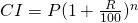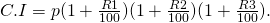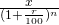# Simple and compound interest

Simple interestP= principal amount
R = rate/unit
T = time/year month day etc.

Compound interestP= principal amount
R= rate/unit
T= time unit

Where rate are difference for different unit or time.

ThanPresent worth of Rs x lac in year hence is given by present worth=